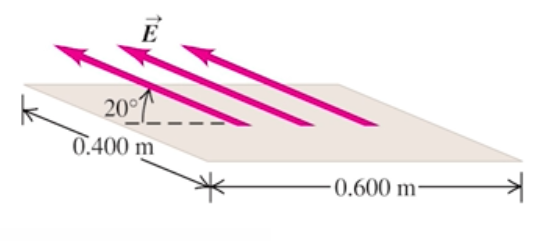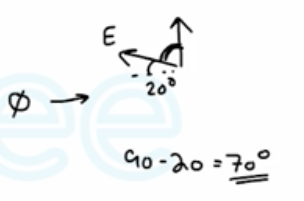Need Help?

Subscribe to Physics 2

###### \${selected_topic_name}
• Notes
• Comments & Questions

A flat sheet is in the shape of a rectangle with sides of lengths 0.400 m and 0.600 m . The sheet is immersed in a uniform
electric field of magnitude 75.0 N/C that is directed at $20^{\circ}$ from the plane of the sheet (Fig. E 22.2 ) . Find the magnitude of the elec-
tric flux through the sheet.$\phi_{E}=E A \cos \theta$$=75 * 0.4*0 .6 * \cos 70$

$=6.16 \mathrm{N} \cdot \mathrm{m}^{2} / \mathrm{C}$

You measure an electric field of $1.25 \times 10^{6} \mathrm{N} / \mathrm{C}$ at a dis-tance of 0.150 m from a point charge. There is no other source of
electric field in the region other than this point charge. (a) What is the electric flux through the surface of a sphere that has this charge
at its center and that has radius 0.150 m? (b) What is the magnitude of this charge?

$\phi_{E}=E A \cos \theta$

$=E A$

$=E4 \pi 1^{2} \longrightarrow \phi_{E} =1.25 * 10^{6}(4 \pi)(0.15)^{2}$

$=3.53 * 10^{5} \mathrm{N} \cdot \mathrm{m}^{2} / \mathrm{c}$

$E=\frac{1}{4 \pi \varepsilon_0} \frac{q}{r^{2}} \longrightarrow q=4 \pi \varepsilon_{0} r^{2} E= 4 \pi \varepsilon_0$

$=\frac{1}{9*10^{9}} (0.15)^{2}\left(1.25*10^{6}\right)$

$=3.13 * 10^{-6} \mathrm{C}$

No comments yet